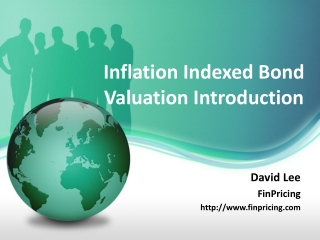DownloadDownload PresentationInflation Indexed Bond Valuation Introduction

# Inflation Indexed Bond Valuation Introduction

Télécharger la présentation## Inflation Indexed Bond Valuation Introduction

- - - - - - - - - - - - - - - - - - - - - - - - - - - E N D - - - - - - - - - - - - - - - - - - - - - - - - - - -
##### Presentation Transcript

1. Inflation Indexed Bond Valuation Introduction David Lee FinPricing http://www.finpricing.com

2. Inflation Bond Summary • Inflation Indexed Bond Introduction • The use of Inflation Indexed Bonds • Valuation • Practical Guide • A Real World Example

3. Inflation Bond Inflation Indexed Bond Introduction • Inflation indexed bonds, also called inflation linked bonds or real return bonds, are bonds where the principal is indexed to a reference inflation index, such as Consumer Price Index (CPI). • The CPI is the proxy for inflation that measures price changes in a basket of goods and services. • The main idea of inflation indexed bonds is that investing in the bond will generate a certain real return. • Inflation indexed bonds pay a periodic coupon that is equal to the product of the daily inflation index and the nominal coupon rate. • Unlike regular (nominal) bonds, inflation indexed bonds assure that your purchasing power is maintained regardless of the future rate of inflation.

4. Inflation Bond The use of Inflation Indexed Bonds • An inflation indexed bond is designed to hedge the inflation risk of the bond. • Since inflation indexed bonds offer investors a very high level of safety, their coupons are typically lower than high-yield bonds. • It is an important vehicle for investors whose liabilities indexed to changes in inflation or wages. • Inflation indexed bonds have favorable performance and lower volatility relative to other risk assets. • It is favorable to retirement planning and pension funds given its inflation protection feature. • Inflation indexed bonds are less liquid than regular bonds.

5. Inflation Bond Valuation • The present value of an inflation indexed bond is given by where • t the valuation date. • the inflation adjusted coupon at payment date . • the inflation adjusted principal at maturity date where P is the principal. • CPI(t) the base reference CPI at time t. • the CPI ratio at where • the issue date. • the discount factor from to t.

6. Inflation Bond Practical Guide • First construct inflation curve by bootstrapping either breakeven inflation swap rates or treasury inflation protected securities (TIPS). • Compute the base reference CPIs at the issue date and each payment date. • Adjust the coupons and principal based on CPI ratio at each payment date. • Discount all the coupons and principal to the valuation date. • The bond price is the sum of all the present values.

7. Inflation Bond A Real World Example